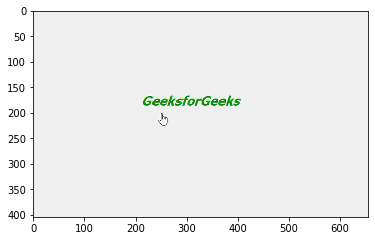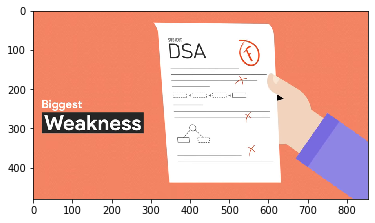# MoviePy – Getting frame from Video File Clip

• Last Updated : 30 Aug, 2020

In this article we will see how we can get the frame at given time from the video file clip in MoviePy. MoviePy is a Python module for video editing, which can be used for basic operations on videos and GIF’s. Combination of frames make the video, at each time there exist a specific frame which is similar to normal image.Getting frame means to get a numpy array representing the RGB picture of the clip at time t or (mono or stereo) value for a sound clip.

In order to do this we will use `get_frame` method with the VideoFileClip object

Syntax : clip.get_frame(n)

Argument : It takes float value as argument

Return : It returns numpy ndarray

Below is the implementation

 `# importing matplotlib``from` `matplotlib ``import` `pyplot as plt`` ` `# importing numpy``import` `numpy as np`` ` `# Import everything needed to edit video clips``from` `moviepy.editor ``import` `*`` ` `# loading video gfg``clip ``=` `VideoFileClip(``"geeks.mp4"``)`` ` `# getting only first 5 seconds``clip ``=` `clip.subclip(``0``, ``5``)``   ` `# getting only first 5 seconds ``clip ``=` `clip.subclip(``0``, ``5``) `` ` `# getting frame at time 3``frame ``=` `clip.get_frame(``3``)`` ` `# showing the frame with the help of matplotlib``plt.imshow(frame, interpolation ``=``'nearest'``)`` ` `# show``plt.show()`

Output :Another example

 `# importing matplotlib``from` `matplotlib ``import` `pyplot as plt`` ` `# importing numpy``import` `numpy as np`` ` `# Import everything needed to edit video clips ``from` `moviepy.editor ``import` `*``   ` `# loading video dsa gfg intro video ``clip ``=` `VideoFileClip(``"dsa_geek.mp4"``) ``    ` `# getting only first 5 seconds``clip ``=` `clip.subclip(``0``, ``5``)`` ` ` ` `# getting frame at time 2``frame ``=` `clip.get_frame(``2``)`` ` `# showing the frame with the help of matplotlib``plt.imshow(frame, interpolation ``=``'nearest'``)`` ` `# show``plt.show()`

Output :My Personal Notes arrow_drop_up